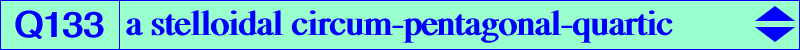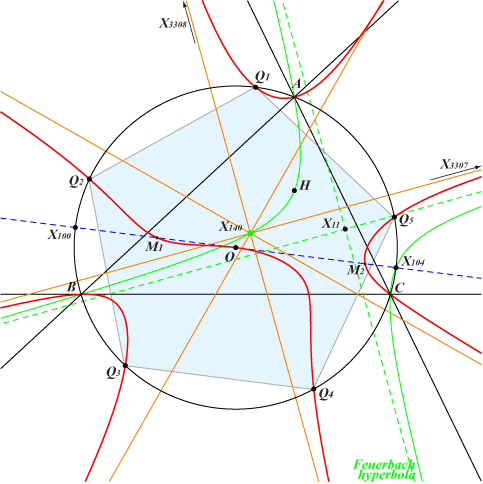too complicated to be written here. Click on the link to download a text file.X(3), X(3307), X(3308) anti-points, see Table 77See Q125 for general explanations where Q133 is Q(X952). See the analogous Q132 = Q(X30), Q134 = Q(X517). The radial center is X(140). The tangent at X(3) is the line X(3)X(8) meeting Q133 again at two points M1, M2 which are isogonal conjugates hence on the McCay cubic K003. Q133 has the remarkable property of meeting the circumcircle at A, B, C and five other points which are the vertices of a regular pentagon.